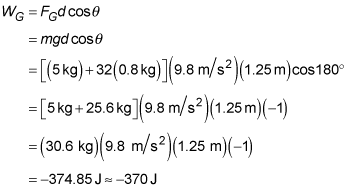##### Physics I: 501 Practice Problems For Dummies (+ Free Online Practice)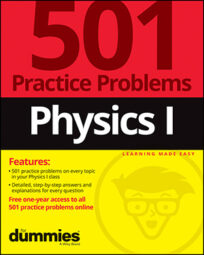Using physics, you can calculate how much work is done when a force is applied in the opposite direction of an object's motion. For example, if you are moving a crate, you can calculate how much work gravity does as you lift the crate off the ground.

When dealing with calculations where the force has a component opposite to the direction of motion, make sure you remember to include minus signs where appropriate!

## Practice questions

1. What is the work done by a westward-directed force of 12.8 newtons that moves an object 3.1 meters to the east?

2. What amount of work does gravity do on a 5-kilogram crate filled with 32 0.8-kilogram lemons if the crate is lifted 1.25 meters off of the ground?

The following are the answers to the practice questions:

1. –40 J

When a force and a distance don't point in the same direction, use the "official" work formula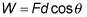where W is the work done on an object, F is the force exerted on the object, d is the distance that the object moves, and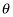is the angle between the direction of the force and the direction of the distance. West and east are separated by 180 degrees, so the work in this situation is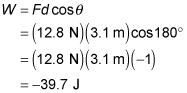2. –370 J

Although gravitational force is present and is essential to solve the problem, you don't need a force diagram because gravity is the only force that concerns you (you don't need to find a net force on the crate). If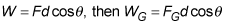where WG is the work done by gravity, FG is the force of gravity, d is the distance that the object moves, andis the angle between the direction of the force (straight down) and the direction of the distance (straight up). The angle between straight up and straight down is 180 degrees. Furthermore, because F = ma, FG = maG. And aG = g near the surface of Earth. Put all that together with the combined mass of the crate and lemons to solve for the amount of work gravity does: